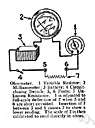# abohm

Also found in: Thesaurus, Medical, Encyclopedia, Wikipedia.

## ab·ohm

(ă-bōm′)
n.
The centimeter-gram-second electromagnetic unit of resistance, equal to one billionth of an ohm.
 Noun 1abohm - a unit of resistance equal to a billionth of an ohmresistance unit - the reciprocal of conductanceohm - a unit of electrical resistance equal to the resistance between two points on a conductor when a potential difference of one volt between them produces a current of one ampere